# Feature Extraction and Classification of Email Spam Detection Using IMTF-IDF+Skip-Thought Vectors

Feature Extraction and Classification of Email Spam Detection Using IMTF-IDF+Skip-Thought Vectors
Dept. of CSE, Neil Gogte Institute of Technology, Osmania University, Hyderabad, Telangana 500007, India

Dept. of CSE, Vasavi College of Engineering, Hyderabad, Telangana 500007, India

Corresponding Author Email:
mokshhyd@gmail.com
Page:
941-948
|
DOI:
https://doi.org/10.18280/isi.270610
8 August 2022
|
Accepted:
18 November 2022
|
Published:
31 December 2022
| Citation

OPEN ACCESS

Abstract:

Spam is a major concern in present emails, and there are several reasons for sending spam emails. The two most common ones are advertising and fraud. If supported by suitable preprocessing approaches, the detection algorithm for spam email or spam classifier will function effectively (removal of noise, removal of stop words, stemming, lemmatization, term frequency). Spam that combines both text and image components is referred to as hybrid spam. Compared to spam emails with images and text, it is more unsafe and complex. To distinguish spam or ham, we must use an effective and smart approach in order to have a strong representation of emails and improve classification performance. In this paper, we propose a multi-modal architecture relying on a feature model (MMA-FM) that concatenates two embedding vectors. The text and image sections of the similar emails were separated using a hybrid model (IMTF-IDF+Skip-thoughts) and the convolutional neural network (CNN) as a feature extraction technique. The extracted features are concatenated and given to Naïve Bayes (NB) and Support Vector Machine (SVM) models to classify hybrid email as either spam or ham. In this paper we used two hybrid datasets: Enron, Dredze, and TREC 2007, which are publicly accessible corpora. Our results show that the SVM model provides an accuracy of 99.16%, which is higher when compared to the Naïve Bayes method.

Keywords:

machine learning algorithms, support vector machine, convolutional neural network, TF-IDF, Skip-thoughts

1. Introduction

Due to how quickly people are using the Internet, which is the easiest way to talk to each other, the number of spam emails is proliferating. Email users spend time deleting spam messages, which occupies large amounts of storage space on the server side and utilizes more network bandwidth, degrading the network's effective transmission speed. Emails are one of the most valuable tools for transferring information and specific ideas, as well as a suggested method for communication in the form of written messages. Emails are shared with a single individual or different sets of individuals at no extra charge. An email is inexpensive to transmit, secure, and deliver without any time delays. Despite these and other benefits, there remains a problem with spam emails [1, 2]. It is inappropriate to send an advertisement that will be broadcast to millions of email subscribers with the hope that at least one will react, regardless of the message. As a result, millions of consumers' email accounts are inundated with spam.

Spam emails cause a lot of issues for the Internet community. Because of spam traffic, servers must wait longer to send real emails. Much research has been done on both approaches, but people still need to discuss the preprocessing method, which was the first step before the email classification process. Noise reduction, stop word removal, stemming, lemmatization, and term frequency are required to detect spam emails. Preprocessing emails enables analysis. These preprocessing steps may affect algorithm performance. Many spam detection studies use different preprocessing methods, which motivates us to do more research. Preprocessing is required before using a classifier to classify spam. Some papers use preprocessing techniques such as cleaning HTML tags and item normalization (currency symbols, email addresses, and URLs) [3-5]. Others use lemmatization, stop-word removal, and case transformation . Noise reduction, removal of stop words , stemming, lemmatization, and term frequency are required to detect spam emails using the Bayesian Classifier and other standard NLP algorithms. Preprocessing emails allows further analysis. Preprocessing steps remove HTML tags, stop words, tokenize text, and count how often words are used . Differences in using existing preprocessing methods urge us to research suitable preprocessing approaches for spam email detection. To achieve high performance in the abovementioned strategies, large-scale data must be available for training.

Deep learning models cannot produce good results on small datasets. To solve this problem, pre-trained models are used. Anti-spam filtering strategies for spam filtering have also been investigated for many years in the domain of machine learning along with cybersecurity fields [9, 10]. These approaches are loosely divided into three different classes, which are text-based algorithms, image-based algorithms, and multi-modal algorithms for spam detection. The first and second classes generally employ an email's textual or image content to filter spam. But the last multi-modal category filters out spam mail by looking at both words and images in an email. Over time, attackers devised new methods to counterfeit existing spam filters, such as image spam (Figure 1). Image spam attacks employ images with text incorporated into them to avoid detection by text-based spam filters. These images make individuals want to click on them, which could lead them to unsafe websites or give them malware. Several strategies for detecting image spam have been developed over the years. Optical Character Recognition (OCR) algorithms extract textual material that can be implemented for image spam detection . To fix this, a method that uses a pre-trained model to detect a feature representation at the sentence level with the help of word vectors is being tested to verify how efficiently it works in the email spam detection job.

## 1.pngFigure 1. Sample spam images

This research also evaluates and compares the effectiveness of multiple machine learning-based classifiers in email filtering for spam messages depending on the specified email header information. This also suggests that important features of the email header will be included for this reason.

The main contribution to this paper is to implement MMA-FM based on IMTF-IDF+Skip-thoughts, CNN, and SVM as a classifier. First, it generates feature vectors from the text and image parts of the same email using the IMTF-IDF+Skip-thoughts and CNN models sequentially. Then, the two generated vectors are concatenated at the feature vector before feeding them into the SVM model for classifying emails as spam or ham. Regarding the text features, adopting the IMTF-IDF+Skip-thoughts method proves its importance in our system by obtaining a highly semantic representation. At the same time, the CNN model also ensures that important features are extracted from the image. Also, concatenating these vectors to feed the SVM classifier improves the performance of the proposed architecture compared to state-of-the-art methods.

2. Related Works

Generally, the accuracy rate of spam detection systems is often affected by the feature extraction techniques adopted. Therefore, to enhance the basic performance value of multimodal spam e-mail systems, more efficient and powerful image-based and text-based feature extraction techniques are required. Below, we describe some of these techniques.

The authors recently proposed a framework which includes an intelligent system with hybrid spam filtering technology  for identifying spam emails by evaluating the headers of the email. Because of its scalability and efficiency, the proposed framework will be appropriate for email servers that are extremely large in size. Their filters can be used independently or in combination with other filters. The email header's extracted features include the following fields: originator, destination, x-mailer, IP address of the sender server, and email topic. Five well-known classifiers were used on the collected features.

Hu et al. , Deepika and Hegde  classified emails based on their titles into four different categories: Sexual, financial, and marketing applications. They mainly focus on the features extracted only from the email header message. They also presented a novel approach for filtering based on classified decision trees (DT), which involves implementation of the Decision Tree methodology to all the categories depending upon the attributes (features) that are collected from the header of e-mail. The retrieved characteristics from the sender’s field are listed as the title of the mail, the date on which it was sent, and the size of the email.

Sheu  suggested an intelligent approach which employs a rule-based technique for detecting the spam found in the header part of the email and syslog’s with the help of comparison with the most common values in the header fields of the specified emails with the server syslog. They found differences between what was in the sent email's header file and what was in the syslog. They used the spamming behavior as a way to describe the sent emails. For the collected features, processing algorithms like rule-based and neural network algorithms like back-propagation were used. With a 0.63% ham misclassification rate, they achieved a 99.6% accuracy rate. Wu  suggested an SVM-based spam discrimination model to classify emails based on email header attributes. With the features they got from email header fields and the SVM classifier, they got a 96.9% recall ratio, a 99.28% precision ratio, and a 98.19% accuracy ratio.

Ye et al.  offered a statistical analysis of junk and legitimate email header session messages, as well as the feasibility of using these messages to conduct spam filtering. The content present in 10024 emails of trash in the mail system was obtained from the database of spam archives and statistically analyzed. The results showed that by using the user agent of mail, email’s message-id, the address of sender, and recipient address as the features, up to 92.5% of spam emails are filtered out.

Despite growing interest in text-based and image-based feature extraction (FE)  strategies for discriminating spam from ham, multimodal solutions that integrate the two FE methods to cope with hybrid spam e-mails are still lacking. Before the classification stage, concatenating image and text characteristics into a single vector gives a strong abstraction to every e-mail.

3. Materials and Methods

We employed four approaches in this paper: Hybrid IMTFIDF+Skipthoughts, CNN, NB, and SVM. Each of these strategies, as well as the proposed multimodal architecture (MMA-FM), are described in this section for detecting hybrid spam e-mails.

3.1 Hybrid model (IMTF-IDF+Skip-thoughts) for text data

The semantic features of words are discarded by conventional techniques like the Bag of Words (BoW) method and the Term Frequency Inverse Document Frequency (TF-IDF) Method.

IMTFIDF Model: The TFIDF states that a phrase has a good differentiating capacity when it occurs in fewer e-mails than when it does not. However, this theory cannot accurately reflect the significance of all terms in practice. The suggested improved TFIDF (IMTFIDF), which is defined as follows:

$\operatorname{IMTFIDF}\left(t_i, d_j, c_k\right)=t f_{i f} \times \log \left(\frac{N}{K_i}\right)$if $\frac{M_i}{M_i+K_i}>70 \%$                      (1)

If ck stands for spam or ham class, N stands for the number of documents per email, tfij is the term frequency of the term ti in e-mail dj of ck. Ki is the number of emails that include the term ti but do not belong to ck. Mi is the total number of the emails that contain the term tiand also belong to ck. If ( $\frac{M_i}{M_i+K_i}$ ) is greater than 70%, the term ti will be used to describe the text properties of this class of e-mail documents.

Skip-thought vector: The skip-thought vector is one of the most significant unsupervised methods used to create sentence embedding. Sentences are to be encoded as fixed-length dense vectors in order to greatly enhance the processing of textual data. The word "embedding," is the representation of words in n-dimensional vector space so that, depending on the training method, semantically related words (such as "boat" and "ship") or semantically similar words (such as "boat" and "water") come closer together, is regarded as an extension of the word embedding. A neural network is used to guess the words around a word and the phrases around a word in a sentence so that a good word vector representation can be made.

## 2.pngFigure 2. Feature extraction from the skip-thought model

A Recurrent Neural Network (RNN) based model is used in the model. The arrangement of both words and sentences is not taken into account by skip-thought vectors. This makes it possible to incorporate rich information. It has been shown that the skip-thought model is successful at learning sentence representations and capturing phrase semantics. As shown in Figure 2, to extract the features, we combine the lookup table (LUT) with the encoder layer. This layer serves as an extractor of features for our spam detection task. The objective is to create a vector that contains a summary of the whole input text. To do this, an encoded vector with one-hot is used to represent each word in the input phrase. With a parameter matrix E, the encoder, which linearly projects onto the one-hot encoded vector wi. This lookup table is used to initialize this matrix. For each input word, a continuous vector is produced via the projection si=Ewi (si is the continuous representation of the word). Now, the series that contains continuous vectors that correspond to the specified words will be transformed into a sequence of sentence-based vectors using an RNN algorithm with GRU activations. For text encoding, we make use of the below expressions:

$r^t=\left(\sigma\left(W_r x^t+U_r h^{t-1}\right)\right)$                      (2)

$z^t=\left(\sigma\left(W_z x^t+U_r h^{t-1}\right)\right)$                      (3)

$\bar{h}^t=\tanh \left(W x^t+U\left(r^t \otimes h^{t-1}\right)\right)$                      (4)

$h^t=\left(1-z^t\right) \otimes h^{t-1}+z^t \otimes \bar{h}^t$                      (5)

here, rt=reset gate, zt=update gate, xt=word embedding, $\bar{h}^t$ = updation of state, tanh = hyperbolic tangent function, W, U, element-wise product, and sigmoid function. In order to extract features with the help of the encoder, we employ two different methods. The unidirectional-skip method and bidirectional-skip methods are used. The front gated recurrent unit (GRU) receives the sentence in the right sequence, while the backward GRU receives the sentence in the reverse order. In order to create a 2,400-dimensional vector, two outputs are added to one another. Combining uni-skip and bi-skip features is what we mean when we talk about combine-skip. These are vectors with 4,800 dimensions. Now that a summary vector of the entire input text is available, our feature vector is this. We provide the machine learning classifier with this feature vector.

Each of the vectors representing one sentence is converted to a skip thought vector and arranged along the rows of the matrices, henceforth keeping the word values filled while keeping the other values as zeroes (sparse matrix). The generated matrix is then combined with the matrix generated using the training phase of the language model. The dot product gives the cosine similarity between the two, thus activating the words that are similar in the context of the combined sentences. Similarly, we evaluate the IMTF-IDF matrix with the language model to get the resultant matrix. The final sentence is thus accumulated using the log likelihood probability of each word from the bag of words, considering n-words (n=3) at a time. Our algorithm requires the input as either integer or float values; thus, we should incorporate one feature extraction layer to convert the words to integers or floats.

3.2 The CNN model for image data

The convolutional neural network (CNN) architecture was developed to solve the problems of the classic cost-related artificial neural network (ANN), time, number of parameters, and selected features. The most important benefits of the CNN model are: extracting the most relevant features, minimizing the number of parameters , training massive data, and decreasing the computation in the network . The CNN model has achieved high performance in a several fields, such as image recognition. It is composed of three main layers: the convolution layer, the pooling layer, and the fully connected layer.

First, the convolution layer is designed to extract features using the convolution operation. The count of feature maps and the specified size of the kernels are two hyperparameters defining the convolution operation. The kernel of a selected size 3×3 or 5×5 is passed in stride over the input tensor. This operation is repeated as many times as the feature map number. The following equation is used to figure out the value of the (m, n) th feature map after the convolution operation is done on the input image f using a kernel h:

$\sum_i \sum_k h(i, j) f(m-j, n-k)$                      (6)

Set N as the size of the input image, K as the size of the kernel, P as the number of layers of zero-padding, and S as the stride size. The size F of the feature map is obtained using the following equation:

$F=1+\frac{N+2 P-K}{S}$                      (7)

Second, the pooling layer consists of reducing the dimension of feature maps and controlling overfitting. It is a required task in the CNN model, and it is located after the convolutional layer. The pooling operation is done by selecting the maximum value in the convolution layer for each region. Third, the last operation in the convolution neural network is the fully connected layer. It is a trainable classifier that takes the pooling layer as input and turns it into one vector with the size that we need. The hidden layer E's activation function is a weighted sum of the input layer, which is given by E=WE. The output layer of the model is coupled with the hidden layer.

Following model training, the specified hidden layer will generate the fixed-size vectors (embedding vectors).

Let Ic,i,j stand for the pixel element in row i and column j of the c image channel; a set of filters K with dimensions k1×k2; and Kc,m,n for the channel c filter weight in row m and column n. The j-th column element in the feature map's row i is as follows.

$(I * K)_{i, j}=f\left(\sum_{m=0}^{k_1-1} \sum_{n=0}^{k_2-1} \sum_{c=1}^c K_{c, m, n} \cdot I_{c, i+m, j+n}\right)$                      (8)

In this paper, the rectified linear unit (ReLU) was used to calculate the activation function f, while C represents the number of channels. The most important information is represented by the maximum value of each region in the feature map, extracted using the max pooling operation. The generated feature maps were then converted into a one-dimensional vector to make a prediction using the softmax activation function. Moreover, batch normalization was adopted in order to prevent over-fitting. The CNN was trained in order to generate the embedding vector of an image using the binary cross-entropy loss function. The trained CNN architecture contained layers from the input to the first dense layer, which had 64 features (neurons). The classification part concatenated the IBOW and the CNN models for the text and image of the same email, the two embedding vectors, to have a rich e-mail representation and improve the classification performance. The n-th text email's embedding vector, Tn, is derived from the model and In is the feature vector of the image of the n-th e-mail, which was generated using the trained CNN model. The concatenation of these two representations, Tn and In produced a vector which was fed to the SVM classifier in order to distinguish between spam and ham.

In this current study, we introduced two efficient algorithms for classification.

Naive Bayes (NB): It is utilized as a baseline classifier  in this work. It uses Bayes' principle using the Poisson process to analyze all data features independently, assuming they are equally important and independent. This classifier is simple and quickly converges.

Support Vector Machine (SVM): It's a supervised machine learning technique for both regression and classification. Every data item in SVM is plotted as a point in n-dimensional space (where n is the number of data features in each sample in the training dataset), and the method attempts to find the best hyperplane that separates the two classes . SVM classifies nonlinearly separable data in a higher-dimensional space with a hyperspace (through a kernel function). SVM is notable for its accuracy and ability to identify huge, nonlinear datasets.

3.3 The proposed approach

The proposed approach MMA-FA filters hybrid email (text and image) which is a binary classification problem consists of three stages: Pre-processing, feature learning or extraction and classification as shown in Figure 3.

## 3.png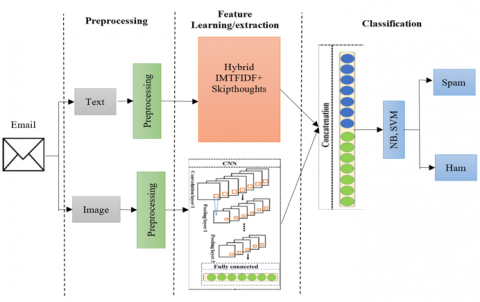Figure 3. The architecture of the proposed MMA-FM model

3.3.1 Dataset

The experiments of the proposed MMA-FA architecture were conducted on two different datasets. We used two publicly available datasets, Enron and Dredze, to build hybrid e-mails. The Enron dataset  contains 17,108 text ham e-mails and 16,537 text spam e-mails, whereas the Dredze dataset  has 2021 personal image ham, 3298 personal image spam, and 16,031 SpamArchive image spam. After removing duplicates from these datasets, they constructed two mixed datasets. The first, which we refer to as Dataset 1, has 600 hybrid ham e-mails (each e-mail contains text and image ham) and 600 hybrid spam e-mails (each e-mail has text and image spam). The second, named Dataset 2, contains 600 hybrid ham e-mails (600 text ham, 300 image ham) and 600 hybrid spam e-mails (600 text spam, 300 image spam).

3.3.2 Data preprocessing

It is required before training a machine-learning model to filter hybrid email for spam or ham classification. Most of the time, removing some noisy or less important keywords can improve classifier performance and reduce the number of dimensions in the feature space. A dataset indeed, but make no mistake; the steps we are taking here to preprocess this data are fully transferable, as illustrated in Figure 4.

Stemming: During this process, various inflected forms of words, such as plurals, gerunds, tenses, and so on, are grouped together. For instance, if we consider words like "group," "groups," and "grouped," which are all synonyms for "group."

Stop words' removal: In the English language, we use certain terms: "a," "and," and "the," which are not required for spam detection. These specified terms are often included, although they don’t provide meaningful information. So, we can remove them, which reduces the feature space and improves classification accuracy.

The preprocessing part consists of cleaning both text and image data. On the one hand, the text data preprocessing step uses a number of methods to improve how well the e-mails are classified. Similarly, the image data preprocessing step consisted of normalizing and resizing these images to 128 × 128 RGB size.

Spelling Error Correction: Error correction may be defined as a word sense disambiguation problem. The objective is then to choose a proper word from a list of confusing words, such as to, too, two, in a certain situation.

## 4.png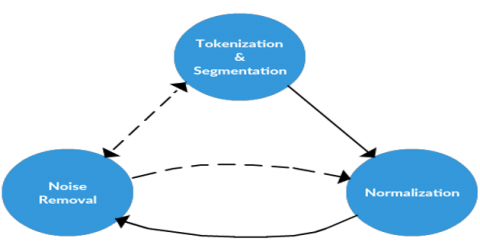Figure 4. State machine of preprocessing phase

3.3.3 Feature learning/extraction

The feature learning part generates the feature vectors from each modality of the email using two models: the IMTF-IDF model and the CNN model for textual and image data, respectively. On the one hand, for the text data, the IBOW model learns and generates the vector representations in a low-dimensional space of a fixed length for each tagged e-mail. Let Ei be the vector representation of an e-mail, which is represented by a one-hot vector; E={E1, E2, E3,.. En} is a set of e-mails, and W is the weight matrix of the model's network connecting the input and the hidden layer. W= K×M, where M is defined as the number of emails and K is the dimension of the hidden layer. In this, E'=WE are the activation function for such hidden layer E. The feature vector for document ID d of the e-mail E'id is constructed from the sentence vector (hidden layer) E' in d dimensions. The hidden layer creates embedding vectors after model training. CNN extracted high-quality features from image e-mails. CNN has three layers: an input layer, numerous convolution layers, and a fully connected layer. CNN model input is 128×128 RGB image in which it employs three convolution layers to extract abstract image features. The rectified linear unit (ReLU) was utilized to construct the activation function f, whereas C indicates the number of channels. The max pooling technique extracts the essential information from each feature map area. The resulting feature maps were transformed into a one-dimensional vector for softmax prediction. CNN was trained to build an image's embedding vector using binary cross-entropy loss.

3.3.4 Classification

The classification phase concatenated the two embedding vectors to optimize classification performance using IMTFIDF+Skipthoughts and CNN models for text and image from the same e-mail. Tn is the IMTFIDF+Skipthoughts model's nth text e-mail embedding vector, while In is the CNN model's nth image feature vector. Concatenating Tn and Increated a vector supplied to the NB and SVM classifiers to identify spam from ham.

4. Results and Discussion

In this experiment, we employ an Intel (TM)-i5 processor with a 3.2GHz CPU clock rate and 8GB of main memory. The feature extraction techniques work with Windows 7 Ultimate, Matlab 2016, and Python 3.5. The percentage of the dataset successfully classified by an algorithm is used to calculate its accuracy. It looks at positives or negatives depending on the situation. Therefore, other criteria for performance evaluation were utilized in addition to accuracy. Also, we use to show the experimental evaluations, include precision, recall, and F1 score metrics.

Accuracy $=\frac{\mathrm{TP}+\mathrm{TN}}{\mathrm{TP}+\mathrm{TN}+\mathrm{FP}+\mathrm{FN}} \times 100 \%$                  (9)

Precision $=\frac{\mathrm{TP}}{\mathrm{TP}+\mathrm{FP}}$                  (10)

Recall $=\frac{\mathrm{TP}}{\mathrm{TP}+\mathrm{FN}}$                  (11)

$\mathrm{F} 1-$ score $=\frac{1}{\text { Precision }}+\frac{1}{\text { Recall }}$                  (12)

TP represents true positive, FP represents false positive, TN is true negative, and FN represents false negative.

RMSE is for the Root of the Mean of the Square of Errors, while MAE stands for the Mean of Absolute Value of Errors. In this context, errors are the disparities between the predicted and actual values of a variable [23, 24]. They are determined as follows:

$R M S E=\sqrt{\frac{\sum\left(x_l-x_m\right)^2}{p}}$                  (13)

$M A E=\frac{\left|\left(x_l-x_m\right)\right|}{p}$                  (14)

where, xl denotes actual value, xm demotes predicted value and p denotes number of observations.

Figure 5 experimental results show that the stop words removal strategy attained the highest accuracy of 0.992 for the SVM related to the Nave Bayes classifier. Also, at a smaller dictionary size of 2000, the SVM achieves 0.965 accuracy, which is a good indication compared to the Nave Bayes classifier.

## 5.png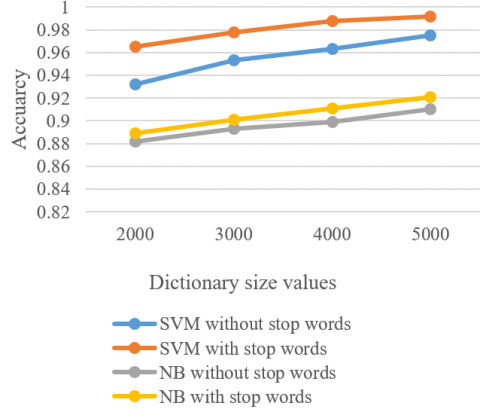Figure 5. Results of experiment on stop words removal preprocessing method

Figure 6 shows that the punctuation of words attained the highest accuracy of 0.982 at a dictionary size of 5000 for the SVM as related to the Nave Bayes classifier. Also, at a smaller dictionary size of 2000, the SVM attains 0.955 accuracy, which is a good indication compared to the Nave Bayes classifier.

## 6.png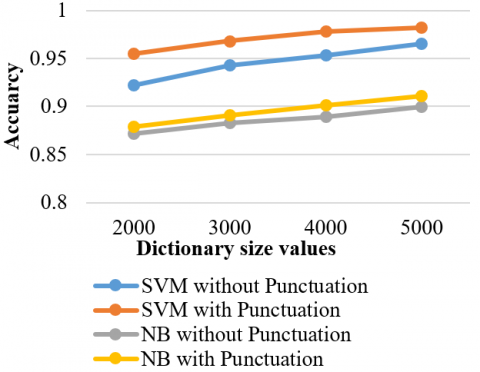Figure 6. Experiment result on stemming and lemmatization preprocessing method

Table 1 list all the four-performance metrics for the Skip thoughts for two models NB and SVM.

Table 1. Performance evaluation of two models with Skip thoughts

 Model Accuracy Precision Recall F1-score Naive Bayes 0.92 0.87 0.83 0.92 SVM 0.98 0.96 0.97 0.97

From Figure 7, it is evident that the Skip-Thoughts approach had some knowledge of word meanings and was able to use this knowledge to deliver the best classification results. When Skip-Thoughts is used on two models, SVM has the highest accuracy of 0.98 compared to the NB model. This is because Nave Bayes can't meet the requirement that features should be independent of each other.

## 7.png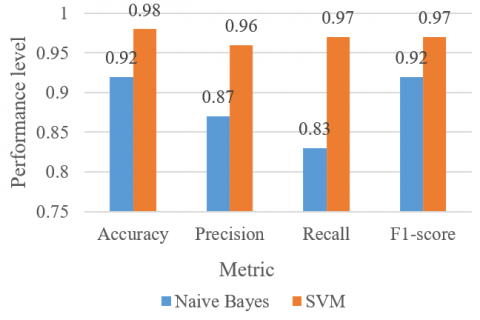Figure 7. Performance comparsion of accuracy, precision, recall and F1-score of Naive Bayes and SVM with Skip thoughts

Table 2 list all the four-performance metrics for the IMTF-IDF for two models NB and SVM.

Table 2. Performance evlaution of two models with IMTF-IDF

 Model Accuracy Precision Recall F1-score Naive Bayes 0.93 0.94 0.91 0.94 SVM 0.96 0.96 0.97 0.96

From Figure 8, it is evident that when given all of the training data, however, the IMTF-IDF concluded that, while knowing what words imply can help with sentiment classification, knowing precise information about the dataset itself was more effective. The IMTF-IDF technique was able to "understand" this dataset-specific information better. When IMTF-IDF is applied to two models, SVM gets the best accuracy of 0.96 in contrast to the Naive Bayes model because it chooses the decision boundary that optimizes the range from the closest data points of all classes (i.e., ham and spam).

## 8.pngFigure 8. Performance comparsion of accuracy, precision, recall and F1-score of NB and SVM with IMTF-IDF

Table 3 list all the four-performance metrics for the combined approach Skip thoughts+ IMTF-IDF for two models NB and SVM.

Table 3. Performance evlaution of two models with Skip thoughts+ IMTF-IDF

 Model Accuracy Precision Recall F1-score Naive Bayes 0.92 0.95 0.93 0.97 SVM 0.99 0.98 0.98 0.98

From Figure 9, it is evident that when we combine the two approaches (i.e., Skip thoughts+ IMTF-IDF), we can see that SVM attains the highest accuracy of 0.99, precision of 0.98, recall of 0.98, and F1-score of 0.98 as compared to the Naive Bayes model. This means that Skip-Thoughts and IMTF-IDF include information about the training set that is complementary.

## 9.png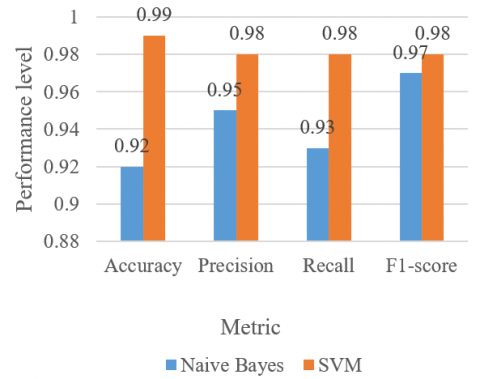Figure 9. Performance comparsion of accuracy, precision, recall and F1-score of NB and SVM with Skip thoughts+ IMTF-IDF

Table 4 lists all the RMSE and MAE errors for two models: Navi Bayes and SVM using Skip thoughts+ IMTF-IDF.

Table 4. RMSE and MAE errors of evaluation of Navi Bayes and SVM

 Model RMSE MAE Naive Bayes 71.2 60.2 SVM 38.6 39.2

## 10.png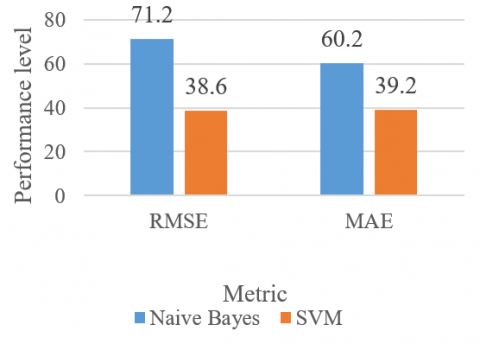Figure 10. Performance comparision of two models for RMSE and MAE errors

Figure 10 shows that RMSE and MAE errors are reduced by Navi Bayes and SVM when using the IMTF-IDF+ Skip-thoughts approach. It is evident that SVM attains a lower RMSE of 38.6 and MAE of 39.2, which is better compared to the Naive Bayes when using the combined approach of Skip thoughts+ IMTF-IDF. Thus, overall, we can interpret that SVM model predictions are correct for Ham and Spam classification as compared to the Naive Bayes model because SVM used hyperparameter tuning to improve the accuracy of the model, whereas NB cannot do that.

5. Conclusions

This paper used a multimodal architecture based on IMTF-IDF+ Skip-thoughts and CNN for hybrid spam e-mail detection. For the proposed classifier, the combination of stop word removal and stemming gives better results than other combinations. However, we also incorporated feature extraction methods with two supervised machine learning classifiers. This proposed multimodal architecture is based on IMTFIDF+Skip thoughts and CNN models for hybrid spam e-mail detection. The IMTF-IDF+Skip-thoughts model was used to generate the feature vector from the text of an e-mail while preserving its semantic features. In contrast, CNN was used as a feature extraction technique to extract the important features from the image of the same e-mail. Finally, the two representations were concatenated and fed to NB and SVM classifiers to distinguish spam from ham, where SVM achieved the best results. The experiments conducted on two different hybrid datasets showed that our proposed architecture is more efficient and outperforms the baseline NB classifier in terms of four metrics: accuracy of 99.16%, precision of 98%, recall of 99.16%, and an F1-score of 98%.

References

 Cheng, T.H., Wei, C.P. (2006). Single-class learning for spam filtering: An ensemble approach. PACIS 2006 Proceedings, 62.

 Fonseca, O., Fazzion, E., Cunha, I., Las-Casas, P.H.B., Guedes, D., Meira, W.,  Chaves, M.H. (2016). Measuring, characterizing, and avoiding spam traffic costs. IEEE Internet Computing, 20(4): 16-24. https://doi.org/10.1109/MIC.2016.53

 Bluszcz, J., Fitisova, D., Hamann, A., Trifonov, A., Jahnichen, P. (2016). Application of support vector machine algorithm in e-mail spam filtering.

 Khan, Z., Qamar, U. (2016). Text mining approach to detect spam in emails. In The International Conference on Innovations in Intelligent Systems and Computing Technologies (ICIISCT2016), p. 45.

 Deepika, M., Hegde, N.P. (2021). Framework for spam detection using multi-objective optimization algorithm. In Smart Computing Techniques and Applications, pp. 345-355. https://doi.org/10.1007/978-981-16-0878-0_34

 Yu, T.Y., Hsu, W.C. (2009). E-mail spam filtering using support vector machines with selection of kernel function parameters. In 2009 Fourth International Conference on Innovative Computing, Information and Control (ICICIC), Kaohsiung, Taiwan, pp. 764-767. https://doi.org/10.1109/ICICIC.2009.184

 Trudgian, D.C. (2004). Spam classification using nearest neighbour techniques. In International Conference on Intelligent Data Engineering and Automated Learning, pp. 578-585. https://doi.org/10.1007/978-3-540-28651-6_85

 Rathod, S.B., Pattewar, T.M. (2015). Content based spam detection in email using Bayesian classifier. In 2015 International Conference on Communications and Signal Processing (ICCSP), pp. 1257-1261. https://doi.org/10.1109/ICCSP.2015.7322709

 Kumar, S., Sharma, R.R. (2014). An empirical analysis of unsolicited commercial e-mail. Paradigm, 18(1): 1-19. https://doi.org/10.1177/0971890714540363

 Swarnalatha, K., Paul, P., Suryansh, D., Akanksha, Manish, A., Singh, S., Jain, S. (2021). Spam detection in twitter data. 2021 IEEE International Conference on Computation System and Information Technology for Sustainable Solutions (CSITSS), pp. 1-3. https://doi.org/10.1109/CSITSS54238.2021.9683387

 Apache spamassassin - open-source anti-spam platform. http://spamassassin.apache.org/. Accessed on 8 Nov., 2019.

 Hu, Y., Guo, C., Ngai, E.W.T., Liu, M., Chen, S. (2010). A scalable intelligent non-content-based spam-filtering framework. Expert Systems with Applications, 37(12): 8557-8565. https://doi.org/10.1016/j.eswa.2010.05.020

 Deepika, M., Hegde, N.P. (2022). Efficient email classification algorithm for better customer support. In Smart Intelligent Computing and Applications, 2: 223-234. https://doi.org/10.1007/978-981-16-9705-0_22

 Sheu, J.J. (2009). An efficient two-phase spam filtering method based on e-mails categorization. International Journal of Network Security, 9: 34-43.

 Wu, C.H. (2009). Behavior-based spam detection using a hybrid method of rule-based techniques and neural networks. Expert systems with Applications, 36(3): 4321-4330. https://doi.org/10.1016/j.eswa.2008.03.002

 Ye, M., Tao, T., Mai, F.J., Cheng, X.H. (2008). A spam discrimination based on mail header feature and SVM. In 2008 4th International Conference on Wireless Communications, Networking and Mobile Computing, Dalian, China, pp. 1-4. https://doi.org/10.1109/WiCom.2008.1139

 Lin, K.Y., Huang, C. (2022). Ensemble learning applications in multiple industries: A review. Inf. Dyn. Appl., 1(1): 44-58. https://doi.org/10.56578/ida010106

 HimaBindu, G., Anuradha, C., Chandra Murty, P.S.R. (2019). Feature extraction techniques in associate with opposition based whale optimization algorithm. Ingénierie des Systèmes d’Information, 24(4): 403-410. https://doi.org/10.18280/isi.240407

 Setianingrum, A., Kalokasari, D., Shofi, I. (2018). Implementasi algoritma multinomial naive bayes classifier. Jurnal teknik informatika, 10. https://doi.org/10.15408/jti.v10i2.6822

 Kashef, R. (2021). A boosted SVM classifier trained by incremental learning and decremental unlearning approach. Expert Systems with Applications, 167: 114-154. https://doi.org/10.1016/j.eswa.2020.114154

 Klimt, B., Yang, Y. (2004). The enron corpus: A new dataset for email classification research. In European conference on machine learning, pp. 217-226. https://doi.org/10.1007/978-3-540-30115-8_22

 Dredze, M., Gevaryahu, R., Elias-Bachrach, A. (2007). Learning fast classifiers for image spam. In CEAS.

 Karunasingha, D.S.K. (2022). Root mean square error or mean absolute error? Use their ratio as well. Information Sciences, 585: 609-629. https://doi.org/10.1016/j.ins.2021.11.036

 Willmott, C.J., Matsuura, K. (2005). Advantages of the mean absolute error (MAE) over the root mean square error (RMSE) in assessing average model performance. Climate Research, 30(1): 79-82. https://doi.org/10.3354/cr030079